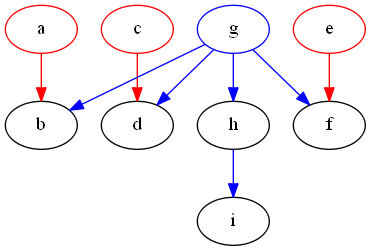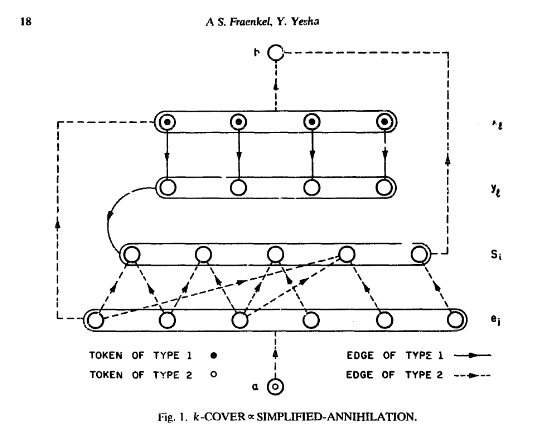Annihilation

I’m going to be out of town for a few weeks, so the next few posts might be delayed from my already slower schedule.

The problem: Annihilation.  This is problem GP9 in the appendix.

The description: G&J’s description is a little obscure, so we’ll go with the one in the paper by Fraenkel and Yesha that has the reduction.

Given a directed graph G=(V, E), and r subsets of E, E1 through Er.  The subsets may not be disjoint, but each edge of E is in at least one subset.

We’re also given r different types of tokens, placed on vertices of the graph.  Each token type corresponds to one of the r subsets of E.  A player moves by taking a token (of type i) and choosing an edge (u,v) in set Ei, where u is the current position of the token.  The token is moved to vertex v in the graph.  If 2 tokens ever meet on the same vertex, both are “annihilated” and removed from the game.  A player loses if they cannot make a move.  Does player 1 have a forced win?  (Though note that the Fraenkel and Yesha paper actually proves whether player 2 has a forced win)

Example: Here is a simple example that will hopefully be useful in the reduction that follows:In this graph, the red edges are in E1 and the blue edges are in E2.  The red vertices currently hold a type 1 token, and the blue vertex holds a type 2 token.

If player 1 makes any move except moving the token on g to the vertex h, player 2 will be able to move a red and a blue token onto the same space, annihilating both.  Then there will be just 2 moves left in the game (the 2 remaining red pieces moving along their edges), the first moved by player 1, the second moved by player 2, then player 1 will have no move and will lose.

If player 1 moves from g->h, then there are 4 more moves in the game (the h->i edge, and the three red edges).  Thus, player 1 will get the last move and will win.

Reduction: Fraenkel and Yesha use Minimum Cover.  I’ll note again here that he reduction will show that the Cover instance is true if and only if player two has a forced win.

So we’re given a collection of sets Si where i goes from 1 to m, and an integer K.  We’re going to build a directed acyclic bipartite graph R= (V, E):

• The graph has vertices xi and yi for i from 1 to K.
• The graph has one vertex for each set Si and one vertex for each element ei in the union of the sets.
• The graph also has two “special” vertices a and b.
• “Type 1” edges go from all xi to its corresponding yi, and from each yi to all Si vertices.
• “Type 2” edges go from a to all ei vertices, from all ei vertices to all set vertices that contain that element, between all e and x vertices, and from all x and S vertices to b.
• Type 1 pieces start on all x vertices.  There is 1 type 2 piece, and it’s on a.

Here is the example used in the paper for the covering problem {{e1,e2}, {e2,e3}, {e3,e4}, {e1,e3,e5}, {e6}} and K=4:An arrow going to a circled group of vertices represents a group of edges going to all vertices in the group.

Notice that without annihilation, the path a type 1 piece takes is from some x vertex to its y vertex, and from there to some S vertex (2 moves), and the path of the type 2 piece is 3 moves (either a->some e-> some x ->b or a->some e->some S ->b), so there is an odd number of moves, and thus player 1 wins if no annihilations happen.  Player 2 wins if a type 1 and type 2 piece collide someplace (on an x or S vertex).

This is because if 2 pieces of different types collide, we remove an odd number of moves from the game:

• If they collide on an x vertex, we remove the 2 moves the type 1 piece can make, and the move the type 2 piece could make from x->b
• If they collide on an S vertex, we remove the one move the type 2 piece makes from S->b

On player 1’s move, they will have to move all pieces off of the x vertices before moving the type 2 token off of the a vertex.  Otherwise, after player 1 moves a->e, player 2 can move e->x (to some x that hasn’t left its starting space yet).

So,  player 1 starts by moving some xi->yi.  Player 2 will move the piece from yi to the next set in the cover.  Recall that there are k different x and y vertices.  So what will happen is that the k vertices that comprise the cover will have tokens on them.

Once all of the type 1 pieces are some S vertex, player 1 will have to move the type 2 piece from a to some e vertex.  If there is a cover, no matter what e vertex player 1 chooses, player 2 will be able to move the token to an S vertex that contains that e element.  If there is no cover, player 1 will be able to choose an e vertex that has no e->S (or e->x) move that causes an annihilation, and player 1 will win.

Difficulty: 7.  This is a very cool reduction, and you can see from the picture how it works.  It’s fun to see how all of the edges and sets work out.